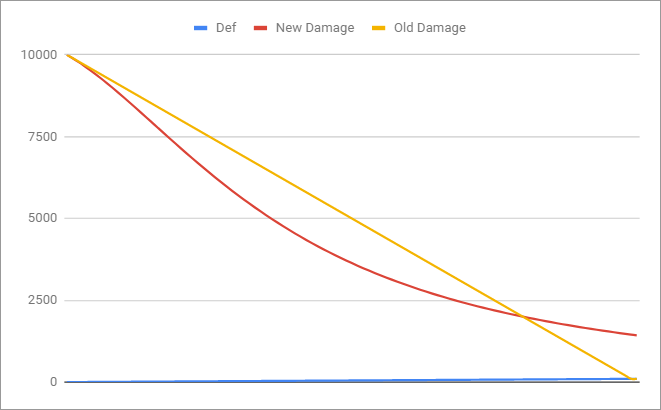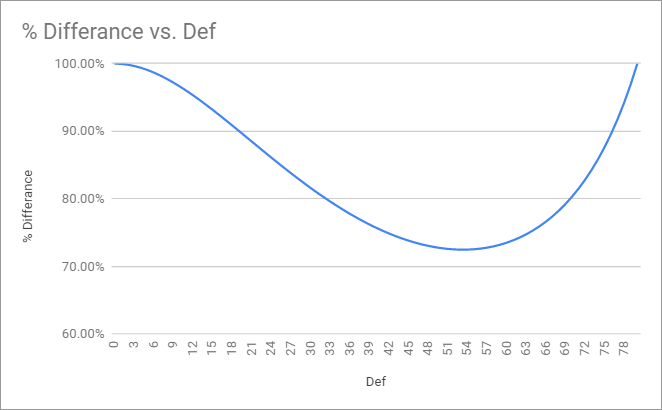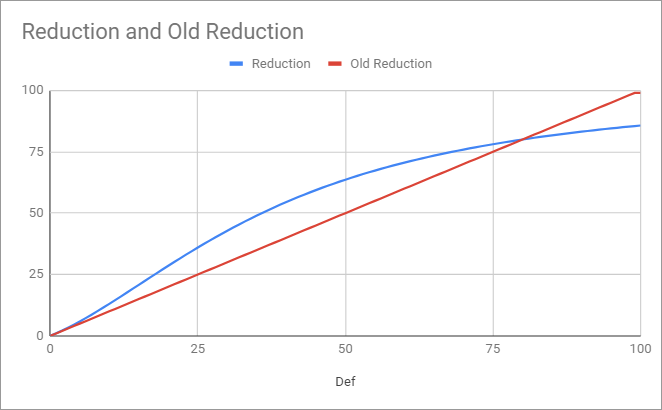# Def / Mdef

## Experimental Changes

These changes are Experimental and can be changes or event reverted at any given time.

### Def

• Remove Def cap of 99
• Damage Formula changed from (Damage * (100 - Def) / 100) -> Damage*100/(100+(Def*(Def/20+1)))
• Crafted bonuses will be applied before the Refine % def
• Refine Increases Gear Def by 1.4% Per safe refine and 2.4% for each refine after (Max 100%)
• Refine Reduces Incoming Damage by 0.40% per safe refine and 0.90% for each refine after (Max 35%)

### Mdef

• Remove Mdef cap of 99
• Damage Formula changed from (Damage * (100 - Mdef) / 100) -> Damage*100/(100+(Mdef*(Mdef/20+1)))
• Ignoring Mdef now ignores Int Mdef

### Knock Down Effects

• Buffs Def/Mdef below 80
• Nerfs Def/Mdef above 80
• Increase Value of damage reduction
• Def/Mdef Ignoring skills are more powerful, ie Misc Skills
• Def/Mdef Reduction is more Valuable, provoke etc
• Crits are more valuable against targets with less then 80 def
• Non Crit Attacks/Skills are slightly weaker (added Def/Mdef Ignore to Onslaught, Mystical and Ranged Mastery to compensate)

### Defense Reversal

• Defense reduced damage (RedDamage) = Damage * (100 / (100 + (HDef * (Hdef / 20 + 1)))
• Vit based damage (VitDamage) = RedDamage * VitDef / 100
• New formula: Final Damage = Damage - ((RedDamage - VitDamage) - Damage)
• Example: Hdef = 50; VitDef = 30; Damage = 10000
```   RedDamage = 3636 = 10000 * (100 / (100 + (50 * (50 / 20 + 1)))
VitDamage = 1090,8 = 3636 * 30 / 100
Final Damage = 17454,8 = 10000 + 7454,8 = 10000 - (-7454,8) = 10000 - (2545,2-10000) = 10000 - ((3636 - 1090,8) - 10000)
Without Ice Pick = 3606
```

### Graphs

Damage New Vs OldDamage Difference New Vs OldDamage Reducution New Vs Old